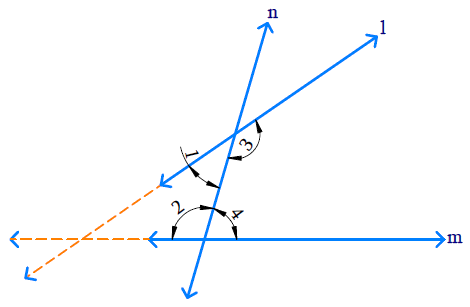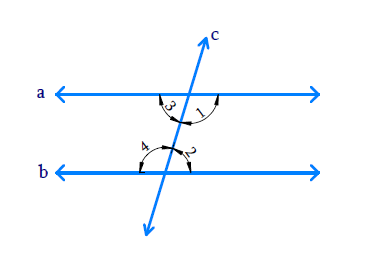# Ex.5.2 Q2 Introduction to Euclids Geometry Solution - NCERT Maths Class 9

Go back to  'Ex.5.2'

## Question

Does Euclid fifth postulate imply the existence of parallel lines? Explain.

Video Solution
Introduction To Euclids Geometry
Ex 5.2 | Question 2

## Text Solution

Reasoning:

Postulate 5 : If a straight line falling on two straight lines makes the interior angles on the same side of it taken together less than two right angles, then the two straight lines, if produced indefinitely, meet on that side on which the sum of angles is less than two right angles.

Steps:

Yes

If ‘$$a$$’ & ‘$$b$$’ two straight lines which are intersected by another line ‘$$c$$’, and the sum of co-interior angles are equal to $$1800$$, then $$a\parallel b$$.According to Euclid’s 5th postulate,

\begin{align} \angle 1 + \angle 2 < 180 ^ { \circ }\end{align}  then \begin{align}\angle 3 + \angle 4 > 180 ^ { \circ } \end{align}

[The interior angles on the same side of two straight lines which are intersected by another line taken together are less than two right angles]

Producing the line ‘$$a$$’ and ‘$$b$$’ further will meet in the side of which is less than $$180 ^ {\circ}.$$

If \begin{align} \angle 1 + \angle 2 = 180 ^ { \circ } \end{align}

then  \begin{align}\angle 3 + \angle 4 = 180 ^ { \circ } \end{align}

The lines ‘$$a$$’ and ‘$$b$$’ do not meet in the side where the angle is lesser than $$180^ {\circ}$$ so they never intersect each other. Hence the two lines are said to be parallel to each other i.e. $$a\parallel b$$Learn from the best math teachers and top your exams

• Live one on one classroom and doubt clearing
• Practice worksheets in and after class for conceptual clarity
• Personalized curriculum to keep up with school